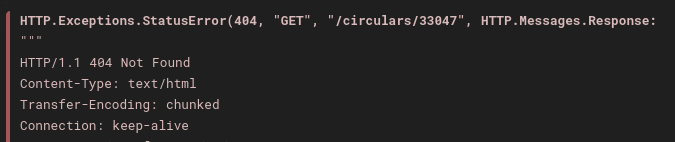# How to handle HTTP.Exceptions.StatusError

I am getting HTTP.Exceptions.StatusError

``````using CSV, DataFrames,HTTP
````````````begin
for x in 33047:33187
url="https://gcn.nasa.gov/circulars/\$x"
txt=String((HTTP.get(url)))
if occursin("report on behalf of the Swift/UVOT team",txt)
hb,he=findfirst(r"^Filter"im,txt)
lr,_=findnext("\n\nThe",txt,he)
cltxt=replace(txt[hb:lr], " +/- "=>"+/-", r"  +(\w)"=>s"\t\1",r"  +(&gt;)"=>s"\t>")
CSV.write("data.csv" ,df)
end
end
end
``````

I tried with `try` and `catch` block but it was not working.

Try in this way:

``````using HTTP
begin
for x in 33047:33187
try
url = "https://gcn.nasa.gov/circulars/\$x"
resp = HTTP.get(url)
@info "processing \$url with status \$(resp.status)"
txt = String(resp)
if occursin("report on behalf of the Swift/UVOT team", txt)
hb, he = findfirst(r"^Filter"im, txt)
lr, _ = findnext("\n\nThe", txt, he)
cltxt = replace(txt[hb:lr], " +/- " => "+/-", r"  +(\w)" => s"\t\1", r"  +(&gt;)" => s"\t>")
CSV.write("data.csv", df)
end
catch e
@error "error at \$x" e
end
end
end
``````

I only show the error in the `catch` branch, not rethrow anything.

1 Like
``````CSV.write("data.csv", df)
``````

this saves only last x output in CSV but i need all with x value in extra column.

I didn’t alter your code in any way - I just handled the error.

CSV overwrites by default. Use
`CSV.write("data.csv",df;append=true)`
to concatenate all x outputs.

You may also have to add `writeheader=true` on the first output (it default to `false` when `append` is `true`)

Hope that helps,

@jdad Yes , How to add extra column for storing GCN circular x value ?

Try adding the statement `df.x=x` just before the CSV.write?

1 Like

Is that `writeheader=true` correct ? code is still running after 15 minutes.``````begin
for x in 33037:33187
try
url = "https://gcn.nasa.gov/circulars/\$x"
resp = HTTP.get(url)
txt = String(resp)
if occursin("report on behalf of the Swift/UVOT team", txt)
hb, he = findfirst(r"^Filter"im, txt)
lr, _ = findnext("\n\nThe", txt, he)
cltxt = replace(txt[hb:lr], " +/- " => "+/-", r"  +(\w)" => s"\t\1", r"  +(&gt;)" => s"\t>")
df.x=x
end
catch e
@error "error at \$x" e
end
end
end
``````

This will write header for every iteration. You could try to replace the one-line-statement CSV.write by a 3-line one

``````if x==33037
CSV.write("data.csv", df)
else
end``````

Note, this suppose that header is the same for every x value. You should check it?

Header isn’t same for every table.Header content is almost same.

Anyway you could write a file for each value of x

`CSV.write("data-\$(x).csv",df)`

1 Like

I got no output. Please see code below:

``````begin
for x in 33037:33187
try
url = "https://gcn.nasa.gov/circulars/\$x"
resp = HTTP.get(url)
txt = String(resp)
if occursin("report on behalf of the Swift/UVOT team", txt)
hb, he = findfirst(r"^Filter"im, txt)
lr, _ = findnext("\n\nThe", txt, he)
cltxt = replace(txt[hb:lr], " +/- " => "+/-", r"  +(\w)" => s"\t\1", r"  +(&gt;)" => s"\t>")
df.x=x
CSV.write("data-\$(x).csv",df)
end
catch e
@error "error at \$x" e
end
end
end
``````

I would suggest using `String(resp.body)` instead of `String(resp)`. In that way you’ll only operate on the extracted HTML string.

Please check if your `occursin("report on behalf of the Swift/UVOT team", txt)` condition is true at least once: I just added a print statement inside that `if` block and and I cannot see a single instance where that condition is true.

So, it is expected to not have any file output - because that code never gets executed.

For x =33211 . It satisfies `occursin` condition but it gives error:

``````ArgumentError
msg

"It is only allowed to pass a vector" ⋯ 60 bytes ⋯ "v` if you want to use broadcasting."
``````

@jdad i can overcome this ArgumentError by removing `df.x=x` from code but `CSV.write("data-\$x.csv",df)` is not saving any file .

Try this code given below:

``````begin
for x in 33110:33511
try
url = "https://gcn.nasa.gov/circulars/\$x"
resp = HTTP.get(url)
txt = String(resp.body)
if occursin("report on behalf of the Swift/UVOT team", txt)
hb, he = findfirst(r"^Filter"im, txt)
lr, _ = findnext("\n\nThe", txt, he)
cltxt = replace(txt[hb:lr], " +/- " => "+/-", r"  +(\w)" => s"\t\1", r"  +(&gt;)" => s"\t>")
@show df
CSV.write("data-\$x.csv",df)
end
catch e
@error "error at \$x" e
end
end
end
``````

This code works for me

``````using HTTP,DataFrames,CSV

function doanalysis()
dfg=nothing
for x in 33037:33287 # was 33037:33187, but mention of 33211 as good candidate
print("\r peeking at \$x ")
try
url = "https://gcn.nasa.gov/circulars/\$x"
resp = HTTP.get(url)
status=resp.status
print(" ",status," "); # println();
if status == 404 ; println("status=",status); continue; end
txt = String(resp.body)
if occursin("report on behalf of the Swift/UVOT team", txt)
println(" Swift report")
# println()
# @info "this is a Swift report"
hb, he = findfirst(r"^Filter"im, txt)
lr, _ = findnext("\n\nThe", txt, he)
cltxt = replace(txt[hb:lr], " +/- " => "+/-", r"  +(\w)" => s"\t\1", r"  +(>)" => s"\t>")
cltxt = replace(cltxt,"&gt;" => "\t>")
# println("cltxt=");print(cltxt)
df.x=[x for i in 1:nrow(df)]
# println();describe(df); show(df);println()
if isnothing(dfg) # x == 33037
dfg=df
else
dfg=vcat(dfg,df)
end # if x is first
CSV.write("data-\$(x).csv",df)
end # if occursin
catch e
println("error ")
# @error "error at \$x" # e
end # trycatch
end # for loop
println()
if !isnothing(dfg)
CSV.write("data-all.csv",dfg)
else
@info "no dfg to write"
end # !isnothing
end # function doanalysis
doanalysis()
# @info "tkumar done"
println("tkumar done.")
``````
2 Likes
• For `if occursin("report on behalf of the Swift/UVOT team", txt)` then also add a column titled Telescope containing SWIFT/UVOT in each row.
• For `if occursin("MASTER-OAFA robotic telescope ", txt)` then also add a column titled Telescope containing Global MASTER-Net in each row.